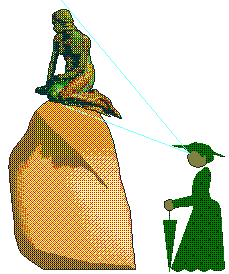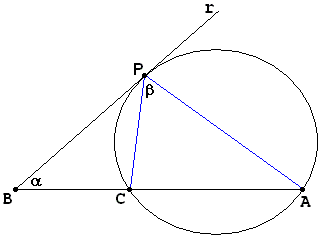Viewing a Statue: the Problem of RegiomontanusElsewhere we established in an indirect manner that for every statue their exists a distance from which it looks its best. We established the fact that such a distance exists without actually supplying means to evaluate it even approximately. Here I'll look into a relevant problem first posed by Regiomontanus (aka Johann Muller, 1436-1476) in 1471 and which is considered as one of the first extremal or optimization problems.

In a sense, for this problem we have a complete constructive solution but only after abstracting its formulation into plain geometric terms. Previously we pondered the question of applicability of abstract mathematical considerations to the real world situations. Now again, by solving an abstract problem, mathematics sheds some light on a real world situation without solving it unambiguously once and for all.This is how we proceed (Ad. Lorsch). First assume that the statue is a vertical rod AB suspended at the height OB from the ground level. Let CP be a horizontal line drawn at the level of viewing (at the level of an observer's eyes.) Draw a circle through A and B tangent to CP at point P and consider the angle APB. We may imagine that point P slides back and forth along the level of viewing. Every position other than the original one takes the point outside the circle. Therefore, for every such position angle APB may only diminish. The only way for it to grow is by placing the point P inside the circle. But this would require our observer to grow in height.

This solves the abstract geometric problem. However, given a real world statue, where should one place the points A and B? Is it possible to satisfactory solve the problem by assuming the statue is flat? Probably not. However, who would claim that the geometric problem has no bearing on the real world one?

Note that in the foregoing discussion we implicitly assumed that the observer's eye level is below the pedestal, i.e. that C is below B. It is edifying to investigate the case where this condition is not observed. If it is not, the sought angle is largest when the observer stands next to the statue at which point, as has been agreed, the statue lack in attractiveness.A variant of the problem has surfaced in the recent times in the problem section of The College Mathematics Journal (March 1998, #624, p. 153) [Diamonds]:Let C be an interior point in the segment AB, and let Z be a variable point on a ray r through B. Suppose r makes angle α with AB, 0 < α < π. Clearly angle β = ∠AZC changes continuously as Z varies on r, and since β is very small when Z is close to B, increases with BZ for a while, and then decreases as BZ gets large, the angle attains a maximum value at some point P on r. Prove that BP is always the same length no matter what the value of α.

The main point here is the same as in the original problem, the extremal angle β is achieved at point P for which the circumcircle of ACP is tangent to r. But, for this point, BP² = BC·AB. So that BC is indeed independent of α.As is often the case, mathematics invented to solve a problem happened to be sufficiently powerful to tackle a more general problem. To what in the real world could the problem just solved be likened? Why, to seeking the distance at which, say, the Leaning Tower of Pisa looks its best.References

1. H. Dorrie, 100 Great Problems Of Elementary Mathematics, Dover Publications, NY, 1965, pp. 369-370
2. R. Honsberger, Ingenuity in Mathematics, MAA, New Math Library, 1970, pp. 89-92
3. R. Honsberger, Mathematical Diamonds, MAA, 2003, pp. 97-98
4. D. Wells, The Penguin Book of Curious and Interesting Puzzles, Penguin Books, 1992, p. 3
5. D. Wells, You are a Mathematician, John Wiley & Sons, 1995, pp. 202-204A Sample of Optimization Problems

• Mathematicians Like to Optimize
• Reshuffling knights - castle defenders
• Isoperimetric Theorem and Inequality
• Fagnano's Problem
• Minimax Principle Demonstration
• Maximum Perimeter Property of the Incircle
• Extremal Problem in a Circular Segment
• Optimization in Four Variables with Two Constraints
• Daniel Dan's Optimization in Three Variables
• Problem in a Special Trapezoid
• Cubic Optimization with Linear Constraints
• Cubic Optimization with Partly Linear Constraints
• Problem M317 from Crux Mathematicorum
• Find the Maximum and Minimum of a Function
• Area of Isosceles Triangle
• Minimum of Cotangents from Saint Petersburg
•